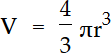Remove from favorites

# Sphere volume formula calculator

Sphere volume formula calculator allows you to find a volume of sphere, by the formula, using radius of sphere.

## Enter the radius of a sphere:Sphere is a perfectly round geometrical object in three-dimensional space that is the surface of a completely round ball. A sphere is defined mathematically as the set of points that are all at the same distance r from a given point.

Formula for volume of a sphere:,
R - radius of a sphere